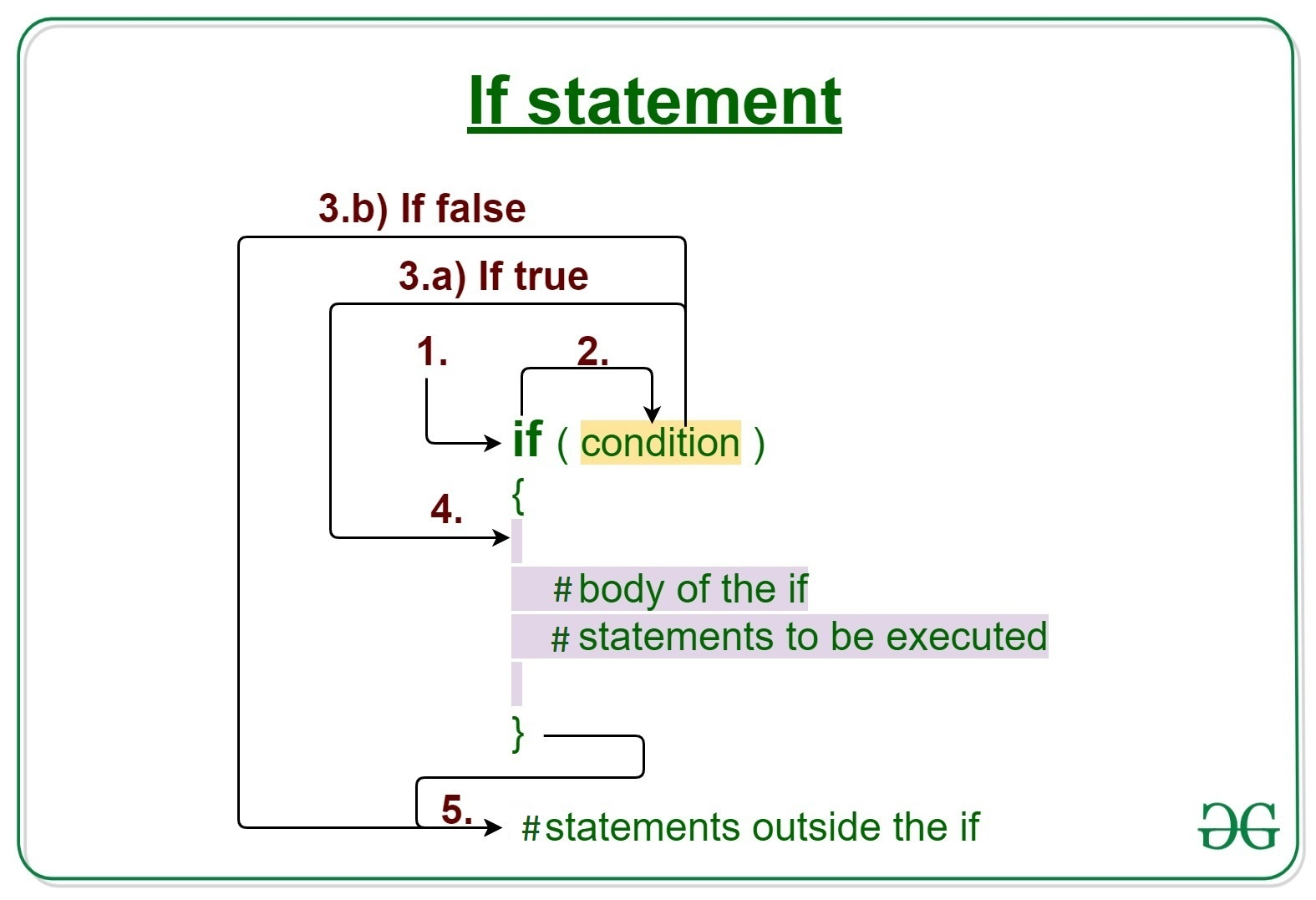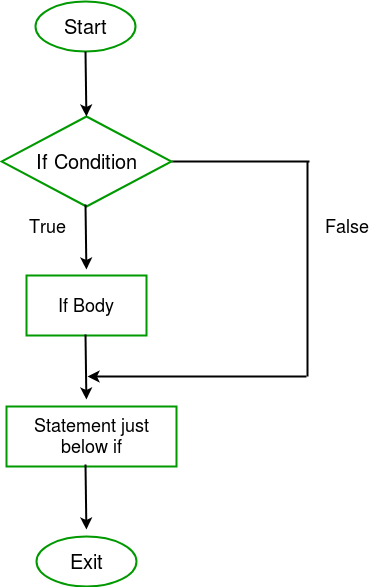GFG App
Open AppBrowser
Continue

If statement is one of the Decision-making statements in the R programming language. It is one of the easiest decision-making statements. It is used to decide whether a certain statement or block of statements will be executed or not i.e if a certain condition is true then a block of statement is executed otherwise not.

Syntax:

```if (expression) {
#statement to execute if condition is true
}```

If the expression is true, the statement gets executed. But if the expression is FALSE, nothing happens. The expression can be a logical/numerical vector, but only the first element is taken into consideration. In the case of numeric vector, zero is taken as FALSE, rest as TRUE.## Working of R Programming if statement

• Control falls into the if block.
• The flow jumps to Condition.
• Condition is tested.
• If Condition yields true, goto Step 4.
• If Condition yields false, goto Step 5.
• The if-block or the body inside the if is executed.
• Flow steps out of the if block.

## Flowchart R Programming if statement## python

 `# R program to illustrate if statement`   `# assigning value to variable a` `a <``-` `5`   `# condition` `if``(a > ``0``)` `{` `    ``print``(``"Positive Number"``)  ``# Statement` `}`

Output:

`Positive Number`

In this example, variable a is assigned a value of 2. The given expression will check if the value of variable a is greater than 0. If the value of a is greater than zero, the print statement will be executed and the output will be “Positive Number”. If the value of a is less than 0, nothing will happen.

## Python

 `# Assigning value to variable x` `x <``-` `12`   `# Condition` `if` `(x > ``20``)` `{` `    ``print``(``"12 is less than 20"``)  ``# Statement` `}` `print``(``"Hello World"``)`

Output:

```12 is less than 20
Hello World```

In this example, variable x is assigned a value. The given expression will check if the value of variable x is greater than 20.

If the value of x is greater than 20, the statement given in the curly braces will be executed and the output will be “12 is less than 20”. Here, we have one more statement outside the curly braces. This statement will be executed whenever we run the program as it’s not a part of the given condition.

## R

 `# R program to illustrate if statement` `# assigning value to variable a` `a <- -5`   `# condition` `if``(a > 0)` `{` `    ``print``(``"Positive Number"``)  ``# Statement` `}``else``{` `    ``print``(``"-ve number"``)` `}`

Output:

`"-ve number"`

My Personal Notes arrow_drop_up